# The Go image/draw package

## Introduction

Package image/draw defines only one operation: drawing a source image onto a destination image, through an optional mask image. This one operation is surprisingly versatile and can perform a number of common image manipulation tasks elegantly and efficiently.

Composition is performed pixel by pixel in the style of the Plan 9 graphics library and the X Render extension. The model is based on the classic “Compositing Digital Images” paper by Porter and Duff, with an additional mask parameter: `dst = (src IN mask) OP dst`. For a fully opaque mask, this reduces to the original Porter-Duff formula: `dst = src OP dst`. In Go, a nil mask image is equivalent to an infinitely sized, fully opaque mask image.

The Porter-Duff paper presented 12 different composition operators, but with an explicit mask, only 2 of these are needed in practice: source-over-destination and source. In Go, these operators are represented by the `Over` and `Src` constants. The `Over` operator performs the natural layering of a source image over a destination image: the change to the destination image is smaller where the source (after masking) is more transparent (that is, has lower alpha). The `Src` operator merely copies the source (after masking) with no regard for the destination image’s original content. For fully opaque source and mask images, the two operators produce the same output, but the `Src` operator is usually faster.

## Geometric Alignment

Composition requires associating destination pixels with source and mask pixels. Obviously, this requires destination, source and mask images, and a composition operator, but it also requires specifying what rectangle of each image to use. Not every drawing should write to the entire destination: when updating an animating image, it is more efficient to only draw the parts of the image that have changed. Not every drawing should read from the entire source: when using a sprite that combines many small images into one large one, only a part of the image is needed. Not every drawing should read from the entire mask: a mask image that collects a font’s glyphs is similar to a sprite. Thus, drawing also needs to know three rectangles, one for each image. Since each rectangle has the same width and height, it suffices to pass a destination rectangle `r` and two points `sp` and `mp`: the source rectangle is equal to `r` translated so that `r.Min` in the destination image aligns with `sp` in the source image, and similarly for `mp`. The effective rectangle is also clipped to each image’s bounds in their respective co-ordinate space.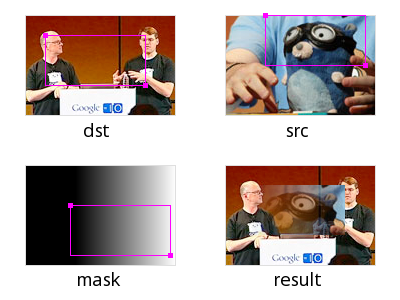The `DrawMask` function takes seven arguments, but an explicit mask and mask-point are usually unnecessary, so the `Draw` function takes five:

``````// Draw calls DrawMask with a nil mask.
func Draw(dst Image, r image.Rectangle, src image.Image, sp image.Point, op Op)
func DrawMask(dst Image, r image.Rectangle, src image.Image, sp image.Point,
mask image.Image, mp image.Point, op Op)
``````

The destination image must be mutable, so the image/draw package defines a `draw.Image` interface which has a `Set` method.

``````type Image interface {
image.Image
Set(x, y int, c color.Color)
}
``````

## Filling a Rectangle

To fill a rectangle with a solid color, use an `image.Uniform` source. The `ColorImage` type re-interprets a `Color` as a practically infinite-sized `Image` of that color. For those familiar with the design of Plan 9’s draw library, there is no need for an explicit “repeat bit” in Go’s slice-based image types; the concept is subsumed by `Uniform`.

``````// image.ZP is the zero point -- the origin.
draw.Draw(dst, r, &image.Uniform{c}, image.ZP, draw.Src)
``````

To initialize a new image to all-blue:

``````m := image.NewRGBA(image.Rect(0, 0, 640, 480))
blue := color.RGBA{0, 0, 255, 255}
draw.Draw(m, m.Bounds(), &image.Uniform{blue}, image.ZP, draw.Src)
``````

To reset an image to transparent (or black, if the destination image’s color model cannot represent transparency), use `image.Transparent`, which is an `image.Uniform`:

``````draw.Draw(m, m.Bounds(), image.Transparent, image.ZP, draw.Src)
``````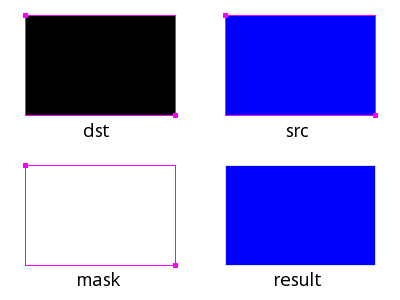## Copying an Image

To copy from a rectangle `sr` in the source image to a rectangle starting at a point `dp` in the destination, convert the source rectangle into the destination image’s co-ordinate space:

``````r := image.Rectangle{dp, dp.Add(sr.Size())}
draw.Draw(dst, r, src, sr.Min, draw.Src)
``````

Alternatively:

``````r := sr.Sub(sr.Min).Add(dp)
draw.Draw(dst, r, src, sr.Min, draw.Src)
``````

To copy the entire source image, use `sr = src.Bounds()`.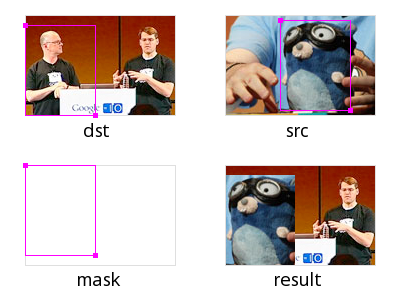## Scrolling an Image

Scrolling an image is just copying an image to itself, with different destination and source rectangles. Overlapping destination and source images are perfectly valid, just as Go’s built-in copy function can handle overlapping destination and source slices. To scroll an image m by 20 pixels:

``````b := m.Bounds()
p := image.Pt(0, 20)
// Note that even though the second argument is b,
// the effective rectangle is smaller due to clipping.
dirtyRect := b.Intersect(image.Rect(b.Min.X, b.Max.Y-20, b.Max.X, b.Max.Y))
``````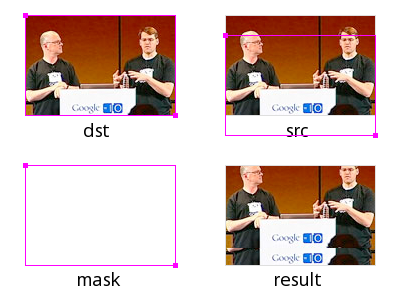## Converting an Image to RGBA

The result of decoding an image format might not be an `image.RGBA`: decoding a GIF results in an `image.Paletted`, decoding a JPEG results in a `ycbcr.YCbCr`, and the result of decoding a PNG depends on the image data. To convert any image to an `image.RGBA`:

``````b := src.Bounds()
m := image.NewRGBA(image.Rect(0, 0, b.Dx(), b.Dy()))
draw.Draw(m, m.Bounds(), src, b.Min, draw.Src)
``````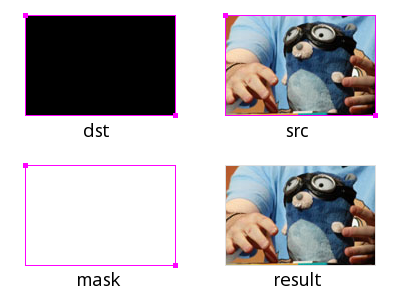To draw an image through a circular mask with center `p` and radius `r`:

``````type circle struct {
p image.Point
r int
}

func (c *circle) ColorModel() color.Model {
return color.AlphaModel
}

func (c *circle) Bounds() image.Rectangle {
return image.Rect(c.p.X-c.r, c.p.Y-c.r, c.p.X+c.r, c.p.Y+c.r)
}

func (c *circle) At(x, y int) color.Color {
xx, yy, rr := float64(x-c.p.X)+0.5, float64(y-c.p.Y)+0.5, float64(c.r)
if xx*xx+yy*yy < rr*rr {
return color.Alpha{255}
}
return color.Alpha{0}
}

draw.DrawMask(dst, dst.Bounds(), src, image.ZP, &circle{p, r}, image.ZP, draw.Over)
``````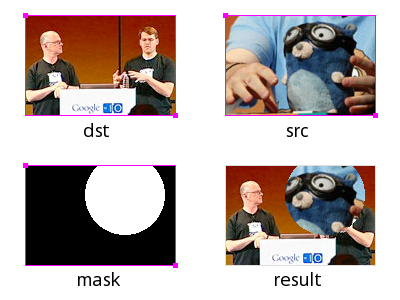## Drawing Font Glyphs

To draw a font glyph in blue starting from a point `p`, draw with an `image.ColorImage` source and an `image.Alpha mask`. For simplicity, we aren’t performing any sub-pixel positioning or rendering, or correcting for a font’s height above a baseline.

``````src := &image.Uniform{color.RGBA{0, 0, 255, 255}}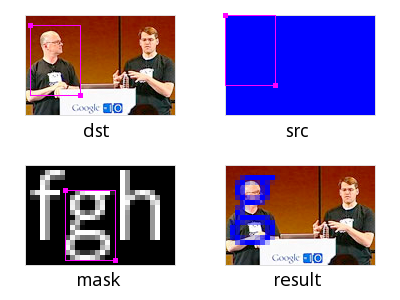The image/draw package implementation demonstrates how to provide an image manipulation function that is both general purpose, yet efficient for common cases. The `DrawMask` function takes arguments of interface types, but immediately makes type assertions that its arguments are of specific struct types, corresponding to common operations like drawing one `image.RGBA` image onto another, or drawing an `image.Alpha` mask (such as a font glyph) onto an `image.RGBA` image. If a type assertion succeeds, that type information is used to run a specialized implementation of the general algorithm. If the assertions fail, the fallback code path uses the generic `At` and `Set` methods. The fast-paths are purely a performance optimization; the resultant destination image is the same either way. In practice, only a small number of special cases are necessary to support typical applications.